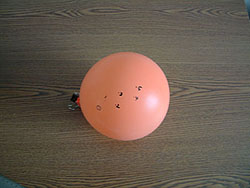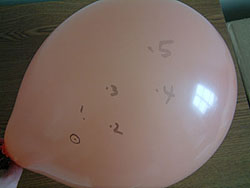In fact, the universe is getting even bigger. Astronomers believe that the universe is expanding – that all points in the universe are getting farther apart all the time. It’s not that stars and galaxies are getting bigger; rather, the space between all objects is expanding with time.

You can make a model of the universe to show how this expansion works. Scientists often use models to help them understand what is happening on a larger scale.Explore 1. Make a model for the expanding universe. Your teacher will divide the class into pairs. He or she will give you and your partner a balloon and a permanent marker. Use the permanent marker to make four to six small dots on one side of the balloon. Don’t put all the dots in a straight line; spread them out a little. Each of these dots represents a galaxy. Now, blow the balloon up slowly, and note what happens to these dots.

Blow up the balloon about halfway. Use the permanent marker to circle one of the outermost dots (the ones farthest to the left or right). This dot represents the Milky Way. Put a piece of paper up to the balloon, and measure the distance from “The Milky Way” to the other “galaxies.” (Your teacher will show you how to make the measurement.) Record your data.Now blow up the balloon all the way. Again, measure the distance from “The Milky Way” to the other galaxies. Record your data. You should now have two columns of data: the distance to each galaxy the first time and the distance to each galaxy the second time.

Use this SkyServer Excel workbook to store all your data and make your calculations.

Explore 2. Calculate the “average speed” of each dot on the balloon with respect to the Milky Way dot. Subtract the distance at the first time from the distance at the second time (d2 – d1). Divide by the amount of time it took you to blow up the balloon (t). If you don’t remember how long it took, just assume a reasonable value, like 5 or 10 seconds.

Calculate the average speed of the dots [(d2-d1)/t]. Record the average speed of each dot as the third column in your workbook.

Explore 3. Use a graphing program such as Excel to graph the second distance on the x-axis and average speed on the y-axis. See SkyServer’s Graphing and Analyzing Data tutorial to learn how to use Excel to graph data. If you don’t have a graphing program, you can use the browser-based Google Sheets.

What does the graph look like? Why do you think the graph has this shape?

As you’ll see in the next activity, your balloon model behaves similarly to the real universe. But like any model, it has its limitations. For one thing, the surface of the balloon (where you drew the dots) is two-dimensional, while the universe is three-dimensional. A three-dimensional model of the universe might be chocolate chip cookie dough rising in an oven. As the dough rises, each of the chips gets farther away from all the other chips. But the cookie dough model has its limitations too – you couldn’t have measured the distances between the chips as easily as you could have with the balloon model.

Can you think of other models for the expanding universe?